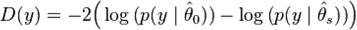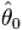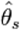# 1.1 - 8.10 - Deviance - Teradata Vantage

## Teradata Vantage™ - Machine Learning Engine Analytic Function Reference

Product
Release Number
1.1
8.10
Published
October 2019
Content Type
Programming Reference
Publication ID
B700-4003-079K
Language
English (United States)

The deviance for a model M 0, based on a data set y, is defined as follows:In the preceding equation:denotes the fitted values of the parameters in the model M 0.denotes the fitted parameters for the full model (or saturated model).

Both sets of fitted values are implicitly functions of the observations y. In this case, the full model is a model with a parameter for every observation so that the data are fitted exactly.

The deviance is used to compare two models—in particular in the case of generalized linear models where it has a similar role to residual variance from ANOVA in linear models (RSS).

Suppose in the framework of the GLM that there are two nested models, M 1 and M 2. In particular, suppose that M 1 contains the parameters in M 2, and k additional parameters. Then, under the null hypothesis that M 2 is the true model, the difference between the deviances for the two models follows an approximate chi-squared distribution with k-degrees of freedom. This provides us an alternative way for computing the log-likelihood ratio of two models.

Deviance is implemented in the GLM function. It computes residual deviance from model deviance and saturated deviance. The function does not compute null deviance.